• Python源码集锦-北京二手房价格数据分析预测
• 北京二手房交易最新消息 二手房交易平台试点发布操作细则，个人出售房源可通过房管部门递交房产证、身份证明原件等进行核验，核验后即可在网络发布;同时，买售双方可将房款存入政府在银行设立的资金监管账户，保证...
• 北京二手房价格预测 项目介绍 根据链家上的北京二手房信息，对数据进行进一步的清洗处理，分析各特征和价格之间的关系，筛选对价格影响比较显著的特征，探索北京二手房的价格情况，并建立房价预测模型 数据预处理 ...
北京二手房价格预测
项目介绍
根据链家上的北京二手房信息，对数据进行进一步的清洗处理，分析各特征和价格之间的关系，筛选对价格影响比较显著的特征，探索北京二手房的价格情况，并建立房价预测模型
数据预处理
读取数据
#导入库
import numpy as np
import pandas as pd
import random
from datetime import datetime
from matplotlib import pyplot as plt
import seaborn as sns
from sklearn.model_selection import train_test_split
from sklearn.preprocessing import PolynomialFeatures
from sklearn.tree import DecisionTreeRegressor
from sklearn.linear_model import Lasso
from sklearn.ensemble import RandomForestRegressor
%matplotlib inline
plt.rcParams['font.sans-serif']=['SimHei']
plt.rcParams['axes.unicode_minus']=False

df=pd.read_csv(r'D:\BI study download\lianjia\lianjia.csv')‘Direction’：房屋朝向 ‘District’：房屋所在区域 ‘Elevator’：电梯情况 ‘Floor’：所在楼层 ‘Garden’：所在楼盘 ‘Id’：房屋id ‘Layout’：房屋房型 ‘Price’：价格 ‘Region’：所在行政区 ‘Renovation’：装修情况 ‘Size’：面积 ‘Year’：所建年份
df.info()观察数据后发现，Elevator有大量空值，其属于分类变量，NAN代表无电梯，因此用无电梯替换内容
df['Elevator'].fillna('无电梯',inplace=True)

删除重复值
df.drop_duplicates(inplace=True)
df.reset_index(drop=True, inplace=True)

数据分析
区域和价格的关系
df.describe()fig,ax = plt.subplots(1,2,figsize=(16,6))
data0.boxplot(column=['Price'], flierprops={'markeredgecolor':'red', 'markersize':4}, ax=ax)
data0.boxplot(column=['Size'], flierprops={'markeredgecolor':'red', 'markersize':4}, ax=ax)经观察数据，北京二手房的面积大概率集中在15-200平米，价格集中在60-1200万。价格最低的二手房为60万，最高6000万；面积最小为15平米，最大为1019平米。北京一套二手房的平均价格为607万，平均面积99平米。 本次分析中，为减少价格极高的二手房对整体数据的影响，只考虑大部分人可以承担的二手房价格，放弃价格属于异常值得数据，即价格超过1200的二手房数据作为异常值删除。¶
df.drop(index = df[df['Price'] > 1200].index, inplace=True)
df.info()可视化不同地区的房屋价格和数量
fig,ax = plt.subplots(2,1,figsize=(30,18))
x = data0['Region'].unique()

y0 = data0.groupby(by=['Region']).size().sort_values(ascending=False)
sns.barplot(x,y0,ax=ax,palette='Reds_d')
ax.set_title('北京各区二手房在售数量')

y1 = data0.groupby(by=['Region'])['Price'].mean().sort_values(ascending=False)
sns.barplot(x,y1,ax=ax,palette='Blues_r')
ax.set_title('北京各区二手房在售均价')北京二手房的分布，东城区最多，其次是西城和朝阳，石景山最少。房价的分布和二手房数量的分布保持一致，也是东城区到石景山从高到低排序。 说明二手房市场价格高的区，房屋供给数量也多。高价地区的房屋均值和和低价地区的价格均值差值较大，说明房屋所在区是影响房间的重要因素。
朝向和价格的关系
plt.figure(figsize=(30,8))
x = df['Direction'].unique()

y = df.groupby(by=['Direction'])['Price'].mean().sort_values(ascending=False)
x1=x[:20]
y1=y[:20]
sns.barplot(x1,y1,palette='Greens_r')南北朝向的二手房的平均价格最高，符合人们挑选房屋时的喜好，因为南北朝向的房屋采光好,但对价格影响相对不大。
装修和价格关系
plt.figure(figsize=(20,8))
x = df['Renovation'].unique()

y = df.groupby(by=['Renovation'])['Price'].mean().sort_values(ascending=False)
sns.barplot(x[:4],y[:4],palette='Reds_r')精装修的二手房价格最高，符合市场规律。而简装和毛坯方的价格十分接近。但影响也不大。
年龄和价格的关系
fig,ax = plt.subplots(2,1,figsize=(30,18))
x = df['age'].unique()

y0 = df.groupby(by=['age']).size().sort_values(ascending=False)
sns.barplot(x,y0,ax=ax,palette='Reds_d')
ax.set_title('北京各房龄二手房在售数量')

y1 = df.groupby(by=['age'])['Price'].mean().sort_values(ascending=False)
sns.barplot(x,y1,ax=ax,palette='Blues_r')
ax.set_title('北京各房龄二手房在售均价')北京二手房市场中的二手房的房龄大部分集中在10-35年，几年新的二手房出售数量较少； 在房屋价格上，几年新的二手房平均价格反而没有10年以上的高。 北京60年以上的老房子也有相对较多出售，而且其价格也不低。¶
房型和价格的关系
#数量和户型的关系
plt.figure(figsize=(20,8))
x = df['Layout'].unique()
print(x)
y = df.groupby(by=['Layout']).size().sort_values(ascending=False)
sns.barplot(x[:10],y[:10],palette='Oranges'plt.figure(figsize=(20,8))
x = df['Layout'].unique()

y = df.groupby(by=['Layout'])['Price'].mean().sort_values(ascending=False)
sns.barplot(x[:10],y[:10],palette='YlGn')北京二手方市场上的房型众多，其中数量最多的是3室1厅的房型，其次是2室1厅。 1室1厅和2室2厅数量接近，而0厅房型的二手房的数量也在前几名。 价格分布基本和房型数量保持一致，说明市场需求大部分集中在3室1厅，2室1厅，1室1厅和2室2厅房型。各房型之间价格有差距，但是差值较小，说明其对价格的影响相对较小。¶
相关性分析
删除无用数据
df1=df.dropna()
df1.drop(columns=['District','Garden','Id','Year','Direction','Layout'],inplace=True)
df1df['Renovation'] = df['Renovation'].map({'毛坯':0,'简装':1,'精装':2,'其他':3})
df['Elevator'] = df['Elevator'].map({'无电梯':0,'有电梯':1})
map1 = {'东城':13, '西城':12, '朝阳':11, '海淀':10, '丰台':9, '昌平':8, '大兴':7, '房山':6, '门头沟':5, '顺义':4,
'亦庄开发区':3, '通州':2, '石景山':1}
df1['Region'] = df1['Region'].map(map1)

df.corr()[['Price']]根据和价格的相关系系数可以看出，面积和价格的关系最大，房屋年龄、有无电梯、楼层的影响较小， 装修方式影响最小。
数据建模
# 分割x，y
x = df.drop(columns=['Price'])
y = df['Price']
x_train, x_test, y_train, y_test = train_test_split(x, y,train_size=0.7)

#训练模型
#决策树
dt = DecisionTreeRegressor(max_depth = 9)
dt.fit(x_train,y_train)
print(round(dt.score(x_train,y_train),2))
print(round(dt.score(x_test,y_test),2))

训练集得分：0.83 测试集得分：0.76
#随机森林
rf = RandomForestRegressor()
rf.fit(x_train,y_train)
print(f'训练集得分：{round(rf.score(x_train,y_train),2)}')
print(f'测试集得分：{round(rf.score(x_test,y_test),2)}')

训练集得分：0.97 测试集得分：0.81
模型评估
from sklearn.metrics import mean_squared_error,explained_variance_score,mean_absolute_error,r2_score
y_dt_train_pred=dt.predict(x_train)
y_dt_test_pred=dt.predict(x_test)
print ("决策树模型评估--训练集：")
print ('训练r^2:',dt2.score(x_train,y_train))
print ('均方差',mean_squared_error(y_train,y_dt_train_pred))
print ('绝对差',mean_absolute_error(y_train,y_dt_train_pred))
print ('解释度',explained_variance_score(y_train,y_dt_train_pred))

print ("决策树模型评估--验证集：")
print ('验证r^2:',dt2.score(x_test,y_test))
print ('均方差',mean_squared_error(y_test,y_dt_test_pred))
print ('绝对差',mean_absolute_error(y_test,y_dt_test_pred))
print ('解释度',explained_variance_score(y_test,y_dt_test_pred))

决策树模型评估–训练集： 训练r^2: 0.8294930763481481 均方差 8860.336245868666 绝对差 67.93515170770496 解释度 0.8294930763481481 决策树模型评估–验证集： 验证r^2: 0.7609894681294798 均方差 12372.514039142869 绝对差 80.04623630560512 解释度 0.7610291620178595
from sklearn.metrics import mean_squared_error,explained_variance_score,mean_absolute_error,r2_score
y_rf_train_pred=rf.predict(x_train)
y_rf_test_pred=rf.predict(x_test)
print ("随机森林模型评估--训练集：")
print ('训练r^2:',rf.score(x_train,y_train))
print ('均方差',mean_squared_error(y_train,y_rf_train_pred))
print ('绝对差',mean_absolute_error(y_train,y_rf_train_pred))
print ('解释度',explained_variance_score(y_train,y_rf_train_pred))

print ("决策树模型评估--验证集：")
print ('验证r^2:',rf.score(x_test,y_test))
print ('均方差',mean_squared_error(y_test,y_rf_test_pred))
print ('绝对差',mean_absolute_error(y_test,y_rf_test_pred))
print ('解释度',explained_variance_score(y_test,y_rf_test_pred))

随机森林模型评估–训练集： 训练r^2: 0.971018612989481 均方差 1506.0082504870181 绝对差 25.9558480075792 解释度 0.9710206214565889 决策树模型评估–验证集： 验证r^2: 0.8149524957794424 均方差 9579.087691070848 绝对差 67.22141777497437 解释度 0.8149560221079576
随机森林模型训练集得分（0.97）和测试集（0.81）得分都高于决策树模型，并且随机森林的均方误差和平均绝对误差都小于决策树。因此随机森林的拟合更好。
实例模拟
一名用户想在二手房市场上出售一套二手房，他的房子情况如下：有电梯（1），6层（6），东城区（13），精装（2），面积100平米（100），房龄20（20），预测其在该数据集统计年份时可在市场上的售价。
apply = np.array([1,6,13,2,100,20]).reshape(1,-1)
#poly_apply = poly.fit_transform(apply)
round(rf.predict(apply),2)

894.66 预测该房屋的价格为894.66万元
展开全文• 2020北京二手房市场半年报精品报告2020.rar
• 2020北京二手房市场半年报精品报告2020.pdf
• 项目数据来源于链家北京二手房数据。 数据预处理 首先导入分析过程中可能运用到的函数包，并读取显示前10行数据。 import pandas as pd import numpy as np import matplotlib.pyplot as plt import matplotlib as ...
目的
本案例得目标主要对数据集中的特征进行量化分析，并且通过图形可视化进行展示出来。项目数据来源于链家北京二手房数据。
数据预处理
首先导入分析过程中可能运用到的函数包，并读取显示前10行数据。
import pandas as pd
import numpy as np
import matplotlib.pyplot as plt
import matplotlib as mpl
import seaborn as sns
from IPython.display import display
%matplotlib inline


前10行数据结果显示如下所示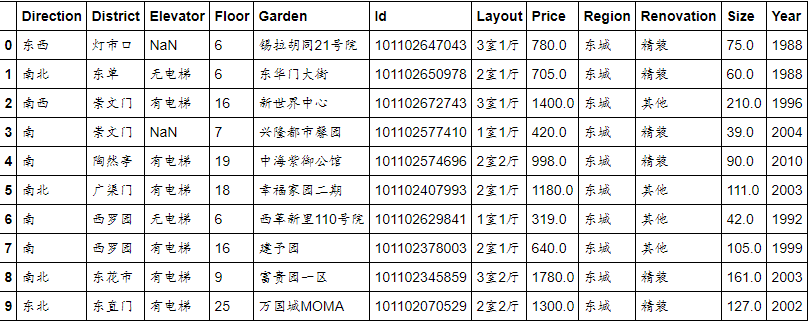然后对数据的基本信息进行查看
lianjia_df.index
lianjia_df.columns
lianjia_df.info()

以上三条代码分别显示如下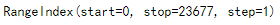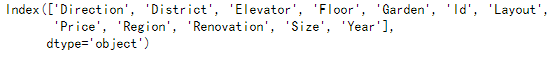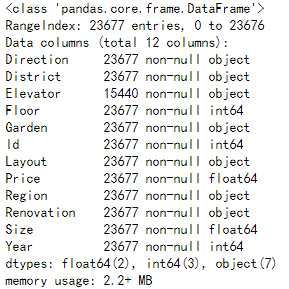再对数据进行描述性统计
lianjia_df.describe()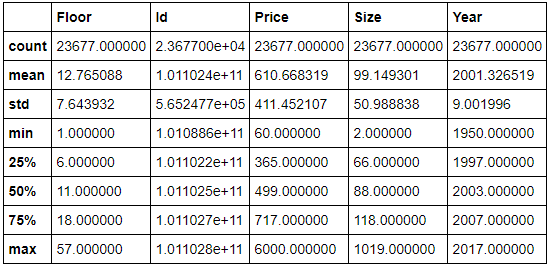新建特征PerPrice，其值等于房价除以面积，并显示前10行数据
df=lianjia_df.copy()
df['PerPrice']=lianjia_df['Price']/lianjia_df['Size']
df[:10]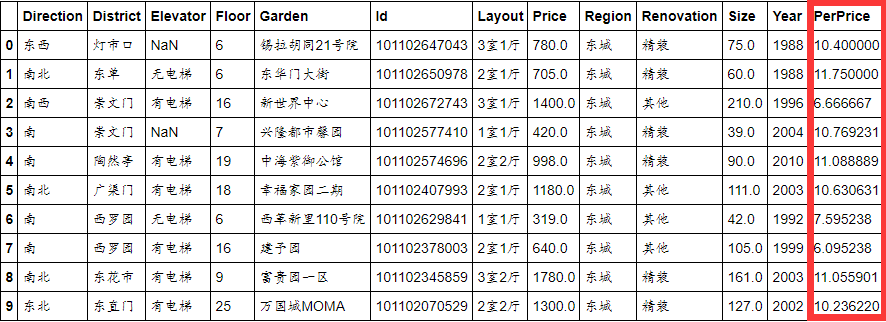对数据的属性重新排列，并将一些无用的属性删除，最后显示前5行数据，代码及结果显示如下
columns=['Region','District','Garden','Layout','Floor','Year','Elevator','Direction','Renovation','PerPrice','Price']
df=pd.DataFrame(df,columns=columns)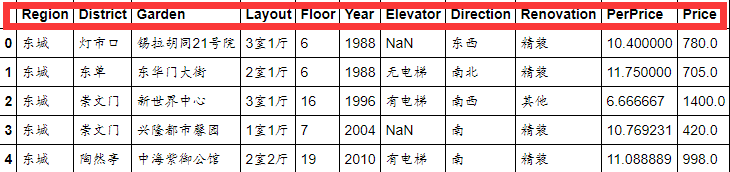对各个地区的价格进行分析
首先按照地区对价格进行分组，其次按照地区对PerPrice进行分组，然后利用柱形图与箱型图进行可视化
df_house_count=df.groupby('Region')['Price'].count().sort_values(ascending=False)
df_house_count=df_house_count.to_frame().reset_index()

df_house_mean=df.groupby('Region')['PerPrice'].mean().sort_values(ascending=False)
df_house_mean=df_house_mean.to_frame().reset_index()

f,[ax1,ax2,ax3]=plt.subplots(3,1,figsize=(20,20))
sns.set(font='SimHei')
m=sns.barplot(x='Region',y='PerPrice',palette='Blues_d',data=df_house_mean,ax=ax1)

"""在柱形图上添加数字"""
for index,row in df_house_mean.iterrows():
m.text(row.name,row.PerPrice,round(row.PerPrice,2),ha='center',color='red',fontsize=20)

ax1.set_title('北京各大区二手房每平米单价')
ax1.set_xlabel('区域')
ax1.set_ylabel('每平米单价')

b=sns.barplot(x='Region',y='Price',palette='Greens_d',data=df_house_count,ax=ax2)

for index,row in df_house_count.iterrows():
b.text(row.name,row.Price,round(row.Price,2),ha='center',color='red',fontsize=20)

ax2.set_title('北京各大区二手房数量对比')
ax2.set_xlabel('区域')
ax2.set_ylabel('数量')

sns.boxplot(x='Region',y='Price',palette='Blues_d',data=df,ax=ax3)
ax3.set_title('北京各大区二手房总价')
ax3.set_xlabel('区域')
ax3.set_ylabel('房屋总价')

plt.show()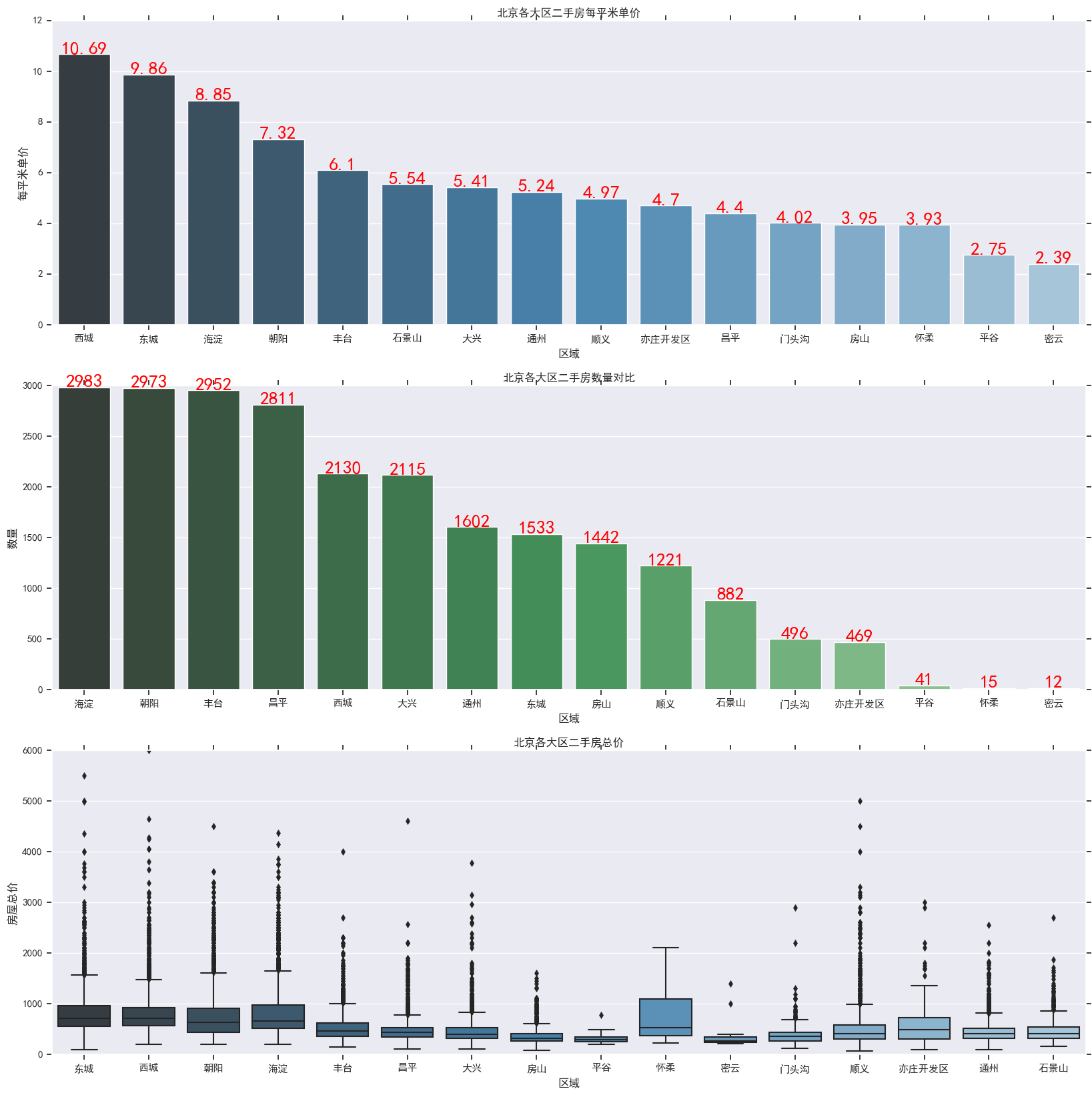Size特征分析
f,[ax1,ax2]=plt.subplots(1,2,figsize=(15,5))
sns.distplot(df["Size"],bins=20,ax=ax1,color='g')
sns.regplot(x='Size',y='Price',data=df,ax=ax2)
plt.show()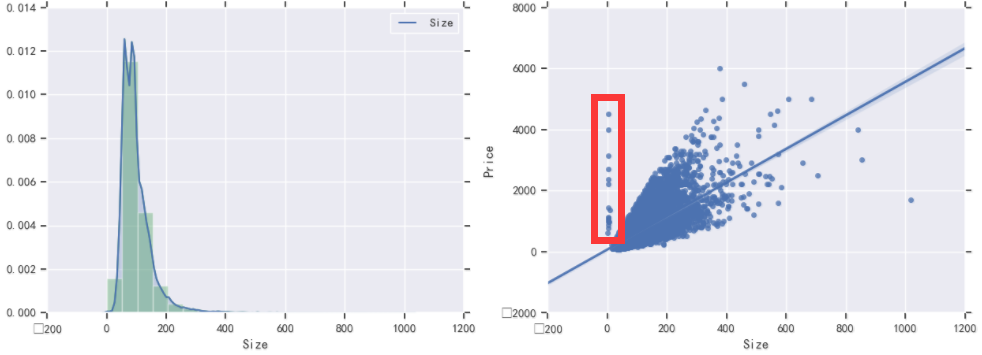在右图中，出现一些离散值，需要将其去除
df=df.loc[(df.Size>10)&(df.Size<1000)]

f,[ax1,ax2]=plt.subplots(1,2,figsize=(15,5))
sns.distplot(df["Size"],bins=20,ax=ax1,color='g')
sns.regplot(x='Size',y='Price',data=df,ax=ax2)
plt.show()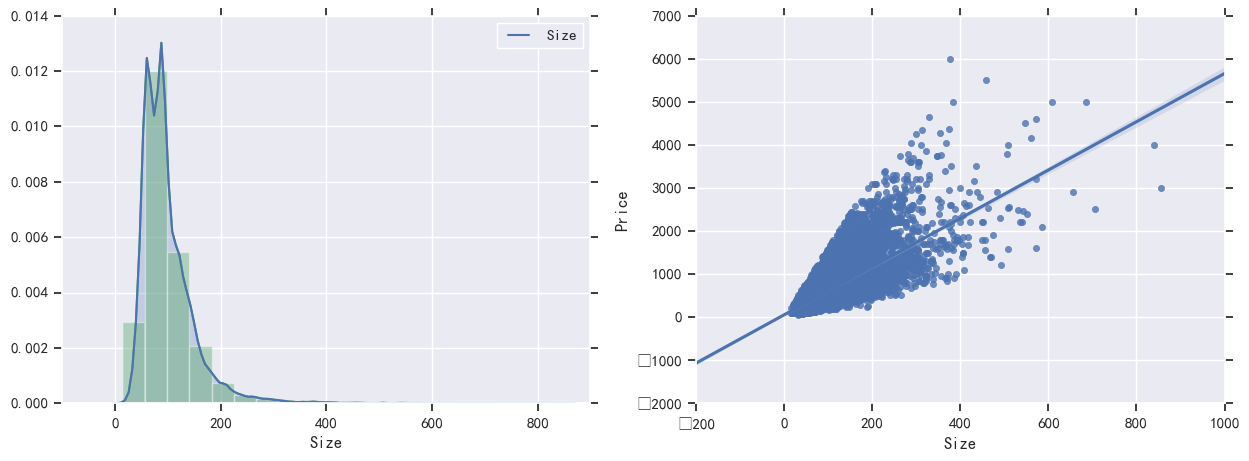处理后的可视化如上图所示
户型分析
f,ax1=plt.subplots(figsize=(20,20))
sns.countplot(y='Layout',data=df,ax=ax1)
ax1.set_title('房屋户型',fontsize=20)
ax1.set_xlabel('数量',fontsize=30)
ax1.set_ylabel('户型')
plt.show()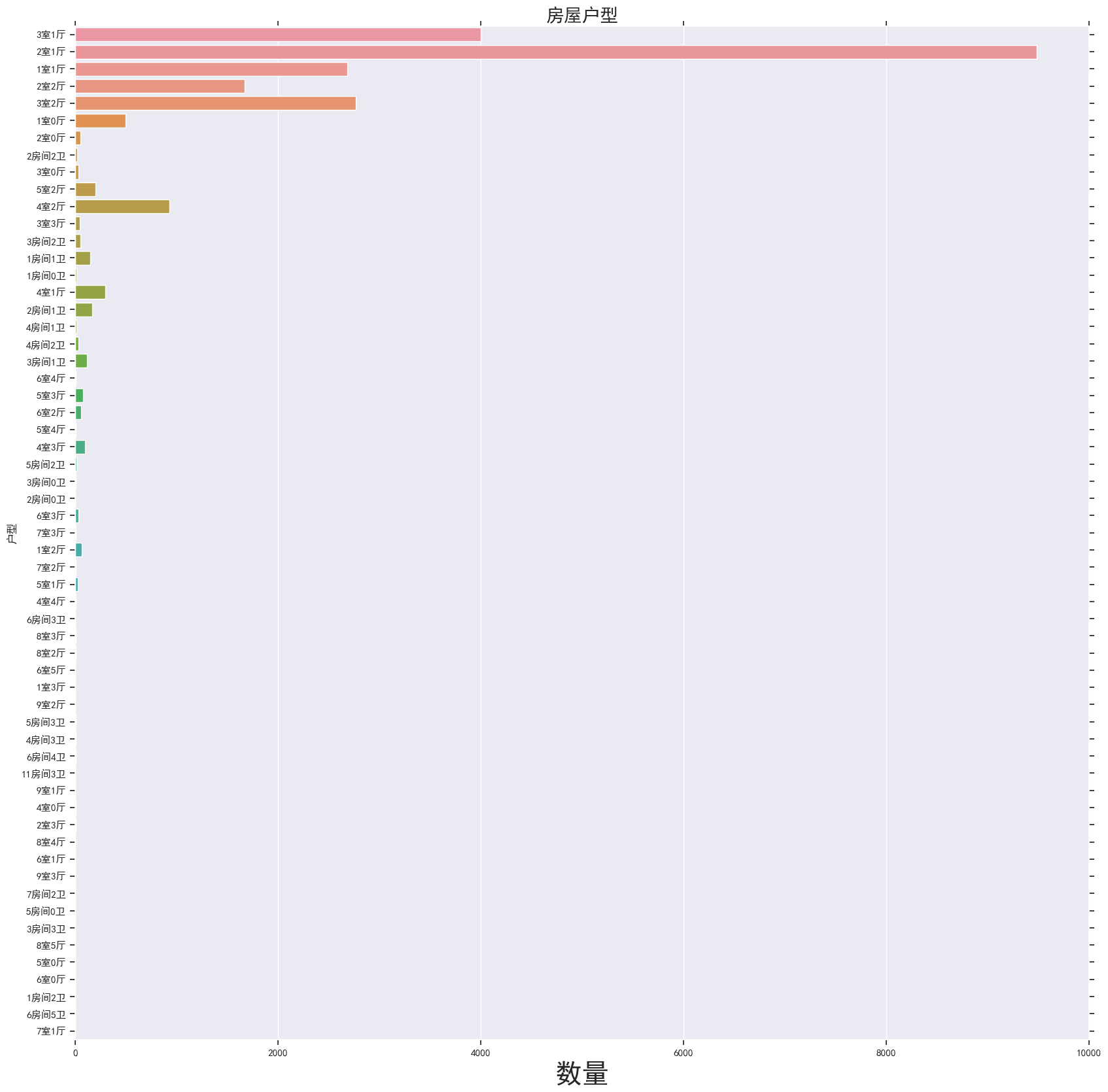Renovation特征分析
df["Renovation"]=df.loc[(df.Renovation!='南北'),'Renovation']

f,[ax1,ax2,ax3]=plt.subplots(1,3,figsize=(20,5))
sns.countplot(df.Renovation,ax=ax1)
sns.barplot(x='Renovation',y='Price',data=df,ax=ax2)
sns.boxplot(x='Renovation',y='Price',data=df,ax=ax3)
plt.show()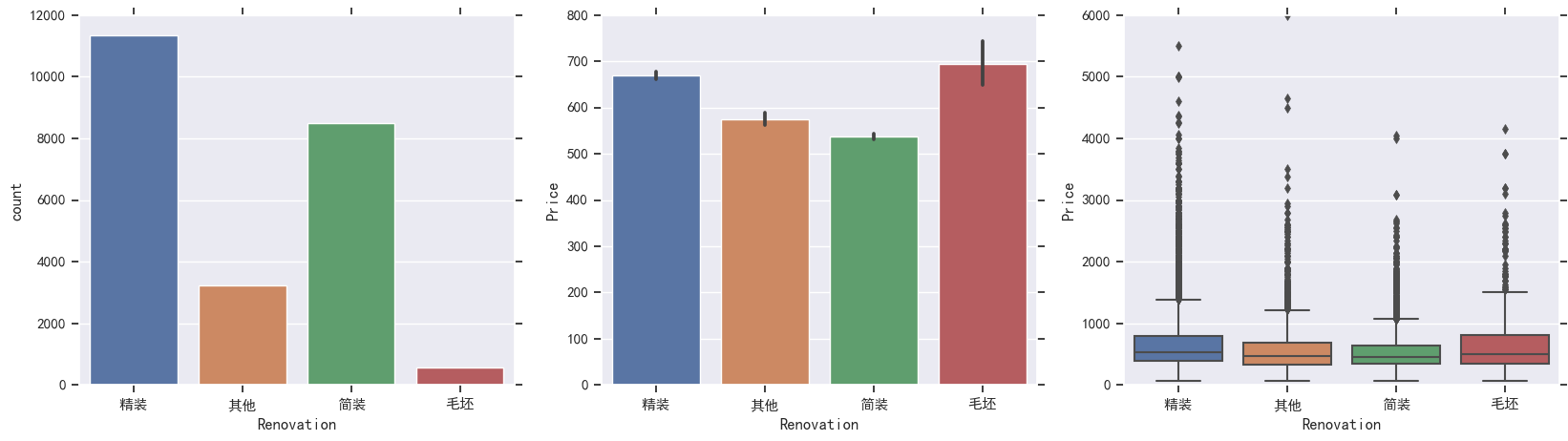对第二个图进行改进后如下代码和结果显示
df4=df.groupby('Renovation')['Price'].mean().to_frame().reset_index()
f,ax2=plt.subplots(figsize=(10,10))
n=sns.barplot(x='Renovation',y='Price',data=df4,ax=ax2)
for ind,row in df4.iterrows():
n.text(row.name,row.Price,round(row.Price,2),ha='center',color='red',fontsize=20)
plt.show()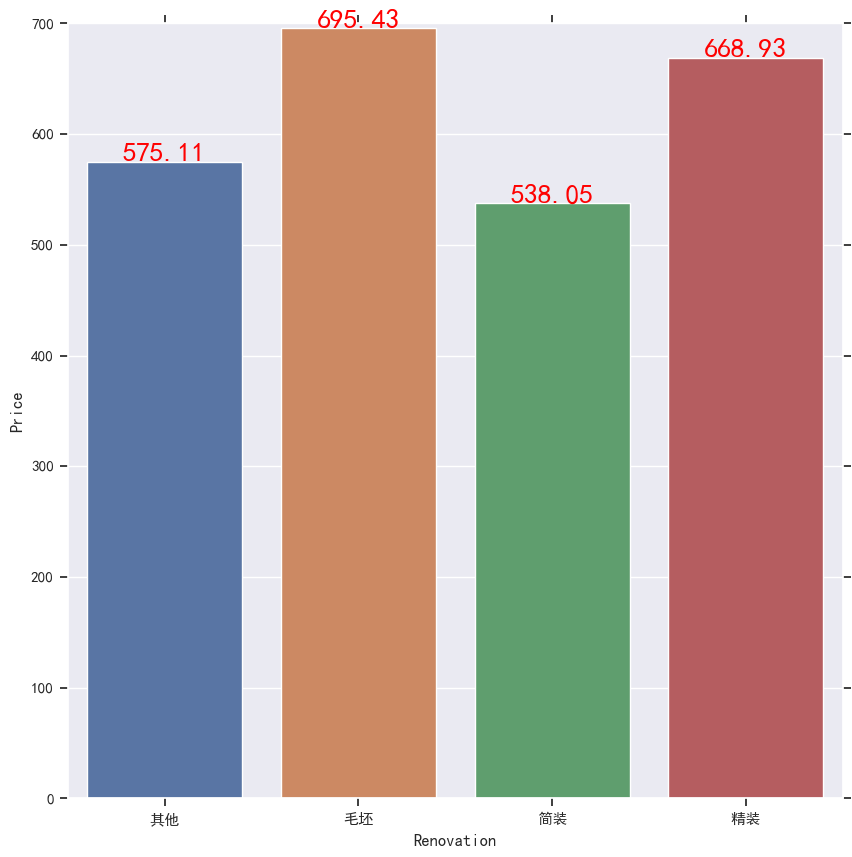Elevator特征分析
df.loc[(df.Floor<=6)&(df.Elevator.isnull()),'Elevator']='无电梯'
df.loc[(df.Floor>6)&(df.Elevator.isnull()),'Elevator']='有电梯'
df5=df.Elevator.value_counts().to_frame().reset_index()

df6=df.groupby('Elevator')['Price'].mean().to_frame().reset_index()

f,[ax1,ax2]=plt.subplots(2,1,figsize=(20,20))
h=sns.barplot(x='index',y='Elevator',data=df5,ax=ax1)
for inde,row in df5.iterrows():
h.text(row.name,row.Elevator,round(row.Elevator,2),ha='center',color='red',fontsize=20)

i=sns.barplot(x='Elevator',y='Price',data=df6,ax=ax2)
for inde,row in df6.iterrows():
i.text(row.name,row.Price,round(row.Price,2),ha='center',color='red',fontsize=20)
plt.show()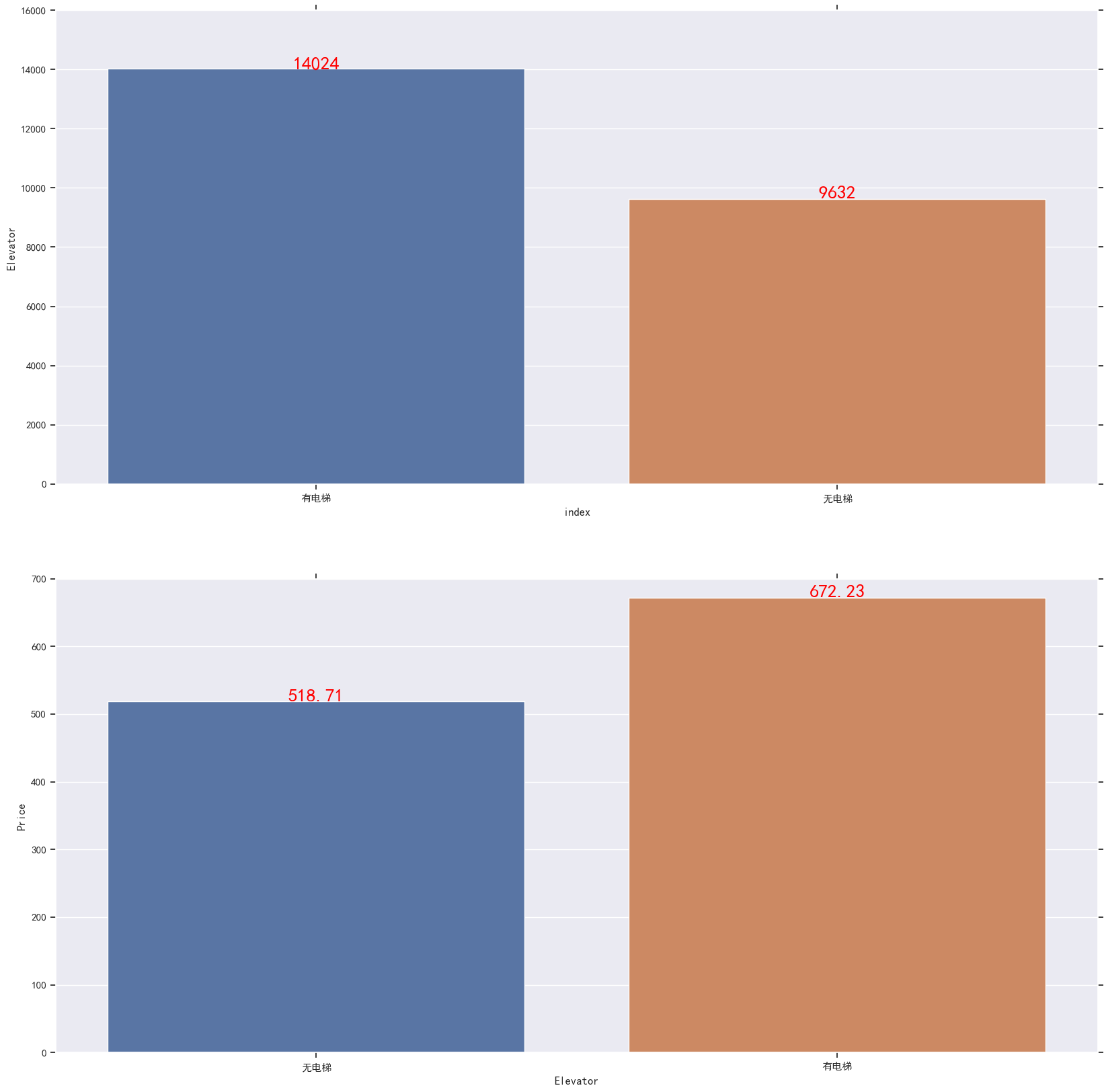Year特征分析
y=sns.FacetGrid(df,row='Elevator',col='Renovation')
y.map(plt.scatter,'Year','Price')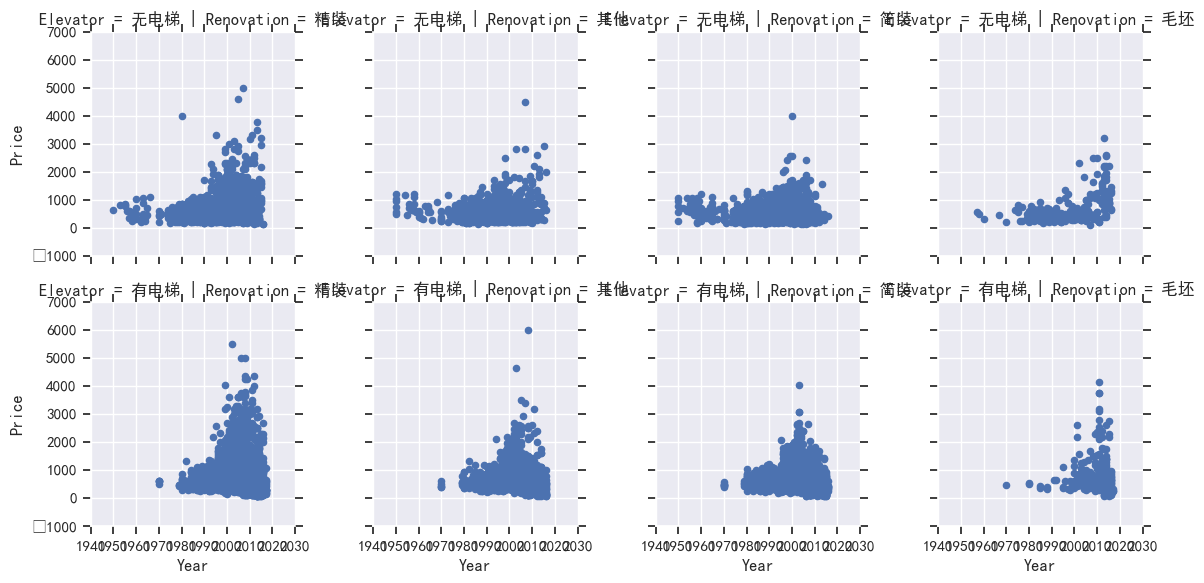Floor特征分析
f,ax1=plt.subplots(figsize=(20,5))
r=sns.countplot(x='Floor',data=df,ax=ax1)
plt.show()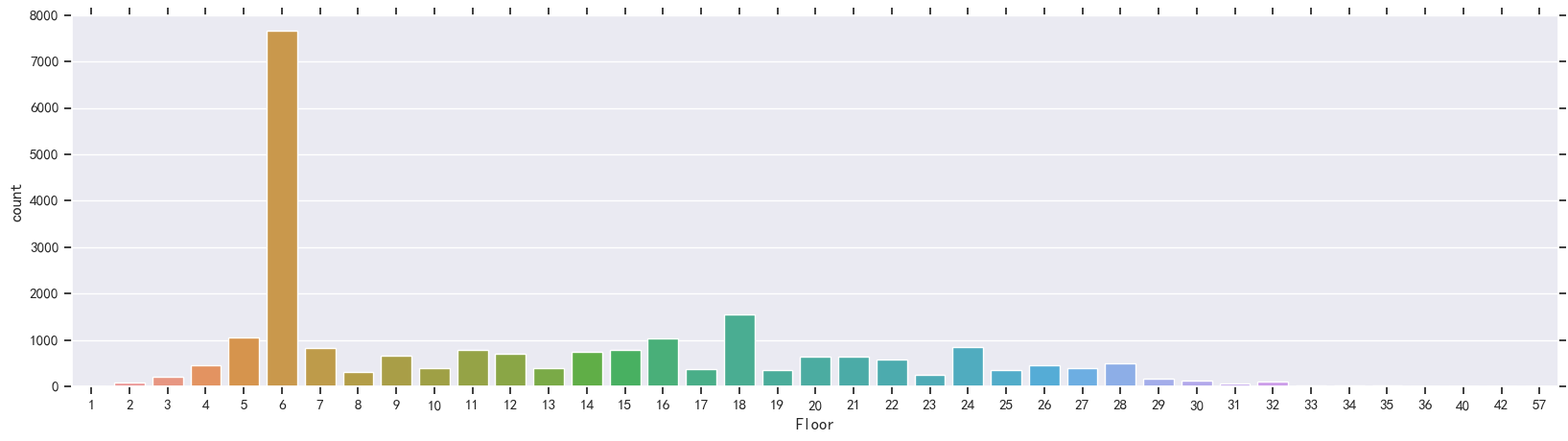展开全文• 北京二手房房价分析与预测目的：本篇给大家介绍一个数据分析的初级项目，目的是通过项目了解如何使用Python进行简单的数据分析。数据源：博主通过爬虫采集的链家全网北京二手房...


北京二手房房价分析与预测

目的：本篇给大家介绍一个数据分析的初级项目，目的是通过项目了解如何使用Python进行简单的数据分析。
数据源：博主通过爬虫采集的链家全网北京二手房数据（关注微信公众号：杰哥的IT之旅，后台回复【二手房数据】添加微信 获取）。
数据初探
首先导入要使用的科学计算包numpy,pandas,可视化matplotlib,seaborn,以及机器学习包sklearn。
import pandas as pd
import numpy as np
import seaborn as sns
import matplotlib as mpl

import matplotlib.pyplot as plt
from IPython.display import display
plt.style.use("fivethirtyeight")
sns.set_style({'font.sans-serif':['simhei','Arial']})
%matplotlib inline

# 检查Python版本
from sys import version_info
if version_info.major != 3:
raise Exception('请使用Python 3 来完成此项目')

然后导入数据，并进行初步的观察，这些观察包括了解数据特征的缺失值，异常值，以及大概的描述性统计。
# 导入链家二手房数据
display(lianjia_df.head(n=2))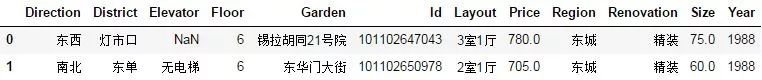初步观察到一共有11个特征变量，Price 在这里是我们的目标变量，然后我们继续深入观察一下。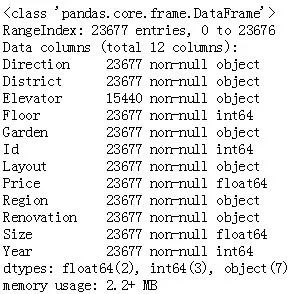23677
Elevator
lianjia_df.describe()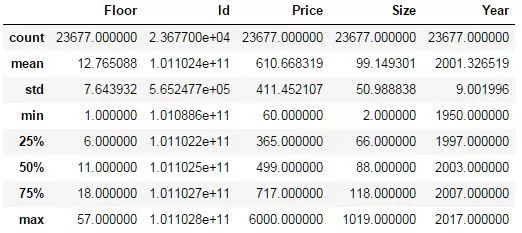上面结果给出了特征值是数值的一些统计值，包括平均数，标准差，中位数，最小值，最大值，25%分位数，75%分位数。这些统计结果简单直接，对于初始了解一个特征好坏非常有用，比如我们观察到 Size 特征 的最大值为1019平米，最小值为2平米，那么我们就要思考这个在实际中是不是存在的，如果不存在没有意义，那么这个数据就是一个异常值，会严重影响模型的性能。
当然，这只是初步观察，后续我们会用数据可视化来清晰的展示，并证实我们的猜测。
# 添加新特征房屋均价
df = lianjia_df.copy()
df['PerPrice'] = lianjia_df['Price']/lianjia_df['Size']

# 重新摆放列位置
columns = ['Region', 'District', 'Garden', 'Layout', 'Floor', 'Year', 'Size', 'Elevator', 'Direction', 'Renovation', 'PerPrice', 'Price']
df = pd.DataFrame(df, columns = columns)

# 重新审视数据集
display(df.head(n=2))

我们发现 Id 特征其实没有什么实际意义，所以将其移除。由于房屋单价分析起来比较方便，简单的使用总价/面积就可得到，所以增加一个新的特征 PerPrice（只用于分析，不是预测特征）。另外，特征的顺序也被调整了一下，看起来比较舒服。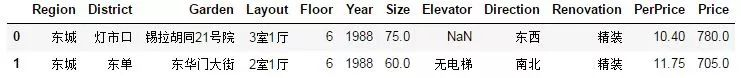数据可视化分析
Region特征分析
对于区域特征，我们可以分析不同区域房价和数量的对比。
# 对二手房区域分组对比二手房数量和每平米房价
df_house_count = df.groupby('Region')['Price'].count().sort_values(ascending=False).to_frame().reset_index()
df_house_mean = df.groupby('Region')['PerPrice'].mean().sort_values(ascending=False).to_frame().reset_index()

f, [ax1,ax2,ax3] = plt.subplots(3,1,figsize=(20,15))
sns.barplot(x='Region', y='PerPrice', palette="Blues_d", data=df_house_mean, ax=ax1)
ax1.set_title('北京各大区二手房每平米单价对比',fontsize=15)
ax1.set_xlabel('区域')
ax1.set_ylabel('每平米单价')

sns.barplot(x='Region', y='Price', palette="Greens_d", data=df_house_count, ax=ax2)
ax2.set_title('北京各大区二手房数量对比',fontsize=15)
ax2.set_xlabel('区域')
ax2.set_ylabel('数量')

sns.boxplot(x='Region', y='Price', data=df, ax=ax3)
ax3.set_title('北京各大区二手房房屋总价',fontsize=15)
ax3.set_xlabel('区域')
ax3.set_ylabel('房屋总价')

plt.show()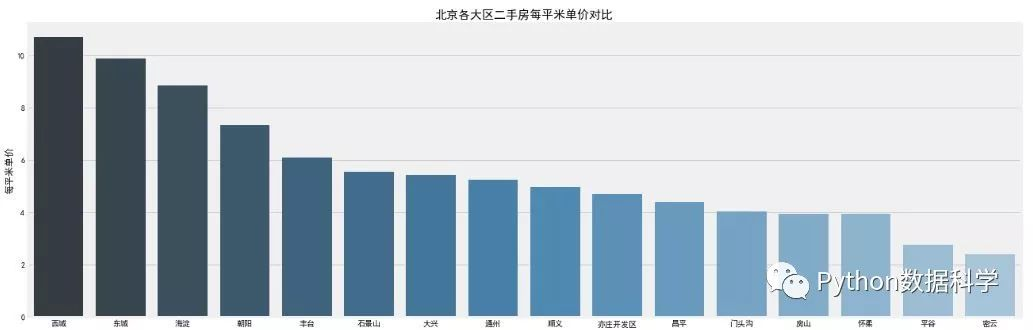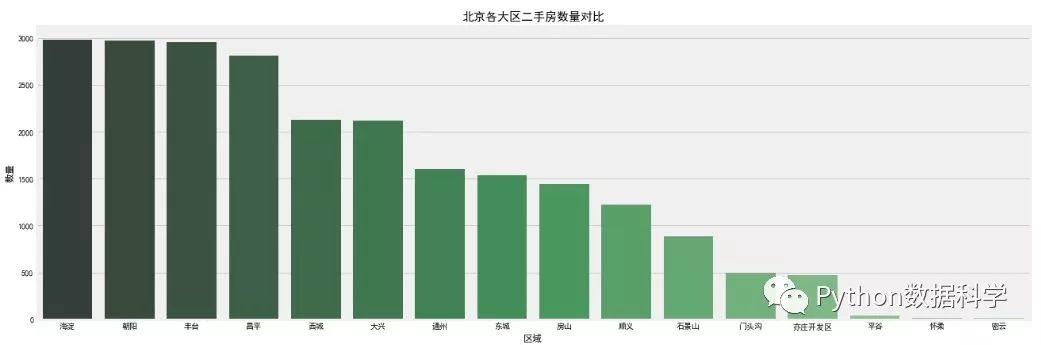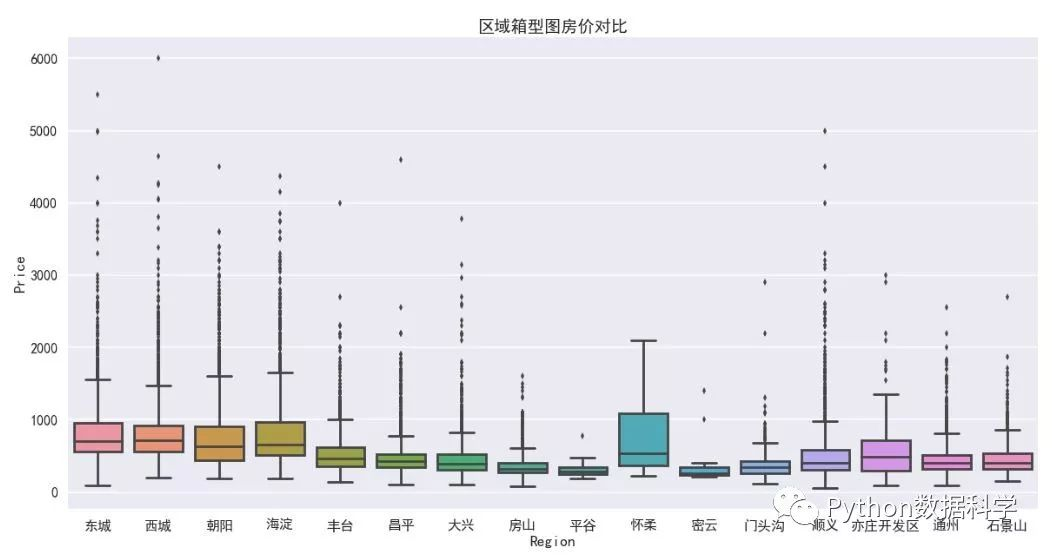使用了pandas的网络透视功能 groupby 分组排序。区域特征可视化直接采用 seaborn 完成，颜色使用调色板 palette 参数，颜色渐变，越浅说明越少，反之越多。
可以观察到：
二手房均价：西城区的房价最贵均价大约11万/平，因为西城在二环以里，且是热门学区房的聚集地。其次是东城大约10万/平，然后是海淀大约8.5万/平，其它均低于8万/平。  二手房房数量：从数量统计上来看，目前二手房市场上比较火热的区域。海淀区和朝阳区二手房数量最多，差不多都接近3000套，毕竟大区，需求量也大。然后是丰台区，近几年正在改造建设，有赶超之势。  二手房总价：通过箱型图看到，各大区域房屋总价中位数都都在1000万以下，且房屋总价离散值较高，西城最高达到了6000万，说明房屋价格特征不是理想的正太分布。
Size特征分析
f, [ax1,ax2] = plt.subplots(1, 2, figsize=(15, 5))
# 建房时间的分布情况
sns.distplot(df['Size'], bins=20, ax=ax1, color='r')
# 建房时间和出售价格的关系
sns.regplot(x='Size', y='Price', data=df, ax=ax2)
plt.show()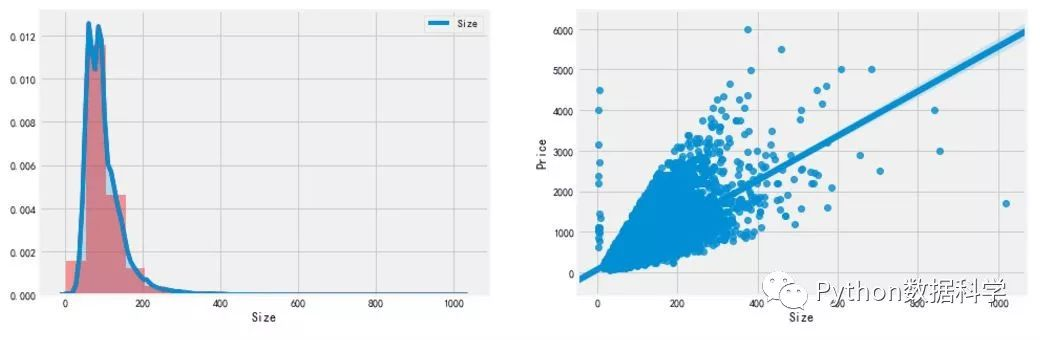Size 分布： 通过 distplot  和 kdeplot 绘制柱状图观察 Size 特征的分布情况，属于长尾类型的分布，这说明了有很多面积很大且超出正常范围的二手房。  Size 与 Price 的关系： 通过 regplot 绘制了 Size 和 Price 之间的散点图，发现 Size 特征基本与Price呈现线性关系，符合基本常识，面积越大，价格越高。但是有两组明显的异常点：1. 面积不到10平米，但是价格超出10000万；2. 一个点面积超过了1000平米，价格很低，需要查看是什么情况。
df.loc[df['Size']&lt; 10]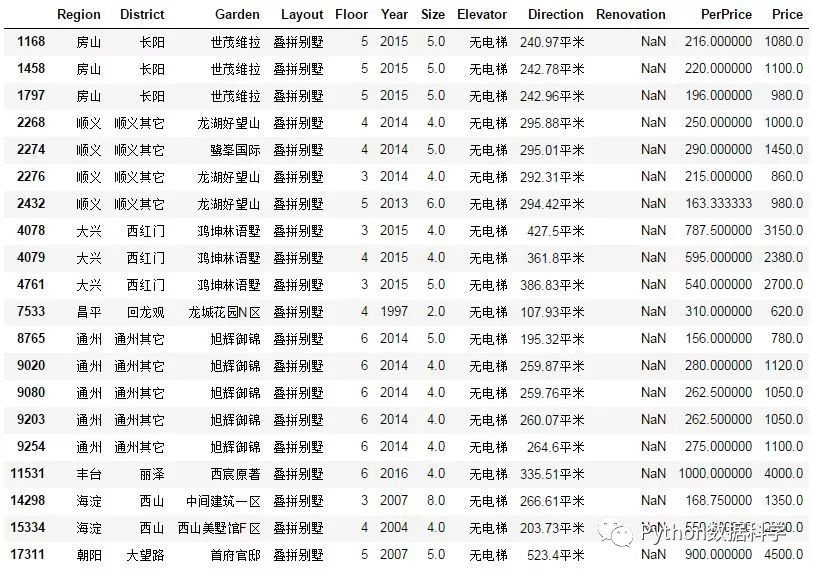经过查看发现这组数据是别墅，出现异常的原因是由于别墅结构比较特殊（无朝向无电梯），字段定义与二手商品房不太一样导致爬虫爬取数据错位。也因别墅类型二手房不在我们的考虑范围之内，故将其移除再次观察Size分布和Price关系。
df.loc[df['Size']&gt;1000]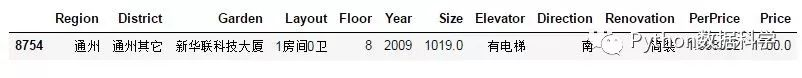经观察这个异常点不是普通的民用二手房，很可能是商用房，所以才有1房间0厅确有如此大超过1000平米的面积，这里选择移除。
df = df[(df['Layout']!='叠拼别墅')&amp;(df['Size']&lt;1000)]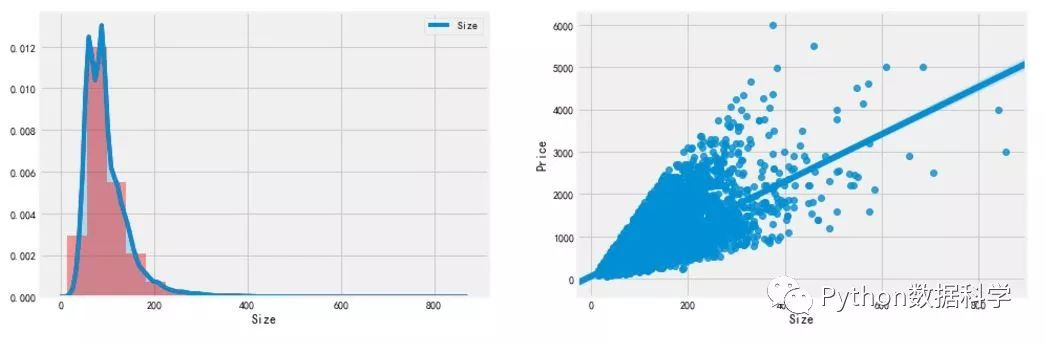重新进行可视化发现就没有明显的异常点了。
Layout特征分析
f, ax1= plt.subplots(figsize=(20,20))
sns.countplot(y='Layout', data=df, ax=ax1)
ax1.set_title('房屋户型',fontsize=15)
ax1.set_xlabel('数量')
ax1.set_ylabel('户型')
plt.show()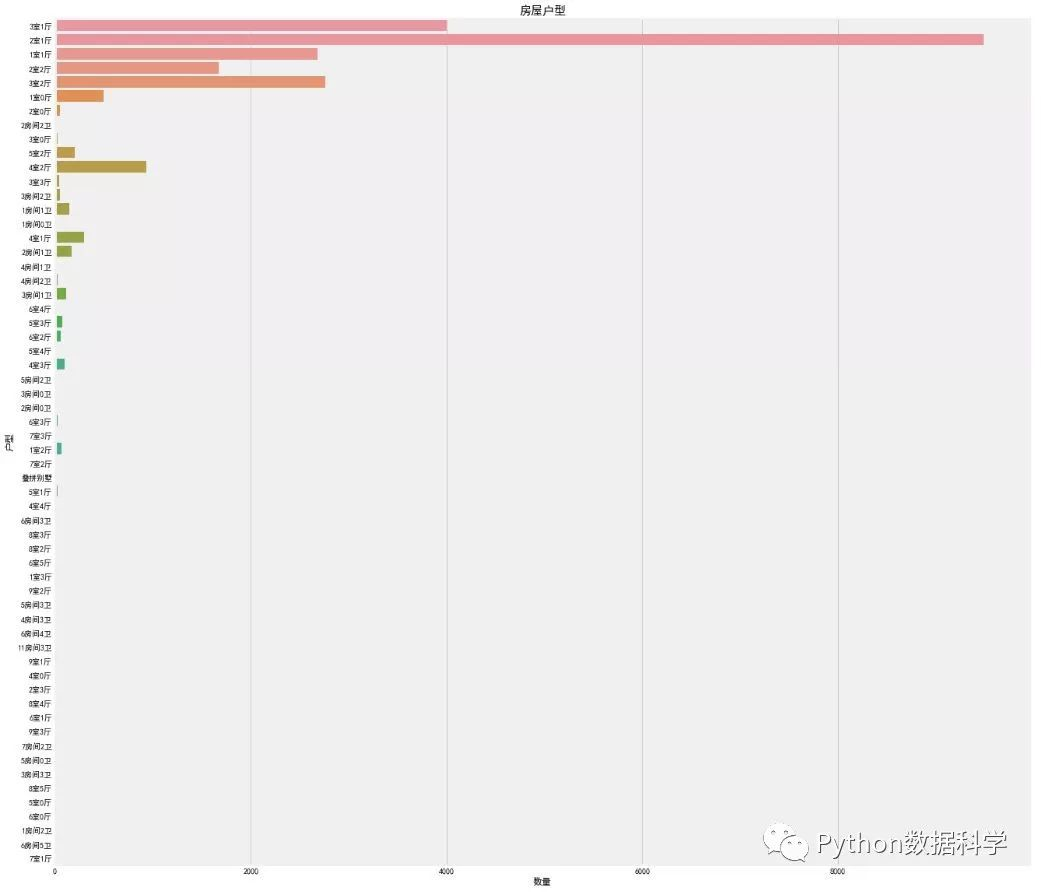这个特征真是不看不知道，各种厅室组合搭配，竟然还有9室3厅，4室0厅等奇怪的结构。其中，2室一厅占绝大部分，其次是3室一厅，2室2厅，3室两厅。但是仔细观察特征分类下有很多不规则的命名，比如2室一厅与2房间1卫，还有别墅，没有统一的叫法。这样的特征肯定是不能作为机器学习模型的数据输入的，需要使用特征工程进行相应的处理。
Renovation 特征分析
发现Renovation装修特征中竟然有南北，它属于朝向的类型，可能是因为爬虫过程中一些信息位置为空，导致“Direction”朝向特征出现在这里，所以需要清除或替换掉。
# 去掉错误数据“南北”，因为爬虫过程中一些信息位置为空，导致“Direction”的特征出现在这里，需要清除或替换
df['Renovation'] = df.loc[(df['Renovation'] != '南北'), 'Renovation']

# 画幅设置
f, [ax1,ax2,ax3] = plt.subplots(1, 3, figsize=(20, 5))
sns.countplot(df['Renovation'], ax=ax1)
sns.barplot(x='Renovation', y='Price', data=df, ax=ax2)
sns.boxplot(x='Renovation', y='Price', data=df, ax=ax3)
plt.show()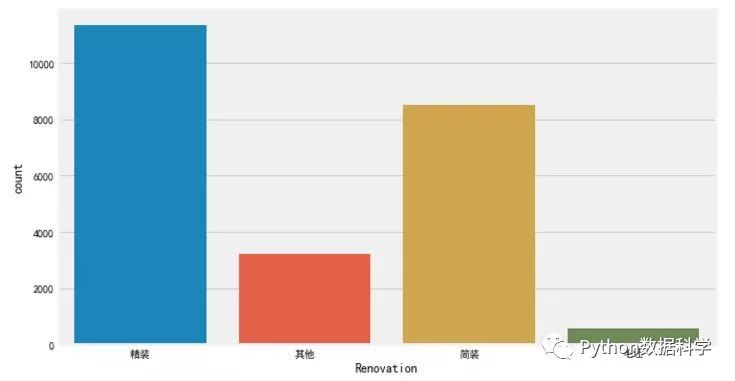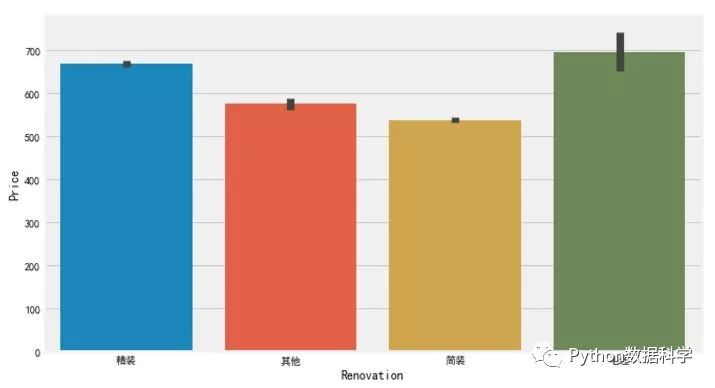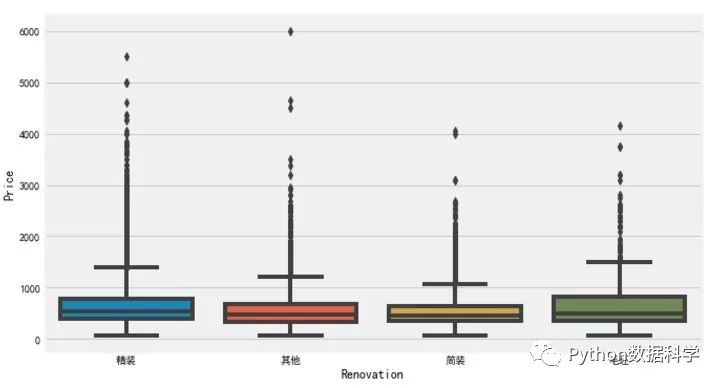观察到，精装修的二手房数量最多，简装其次，也是我们平日常见的。而对于价格来说，毛坯类型却是最高，其次是精装修。
Elevator 特征分析
初探数据的时候，我们发现 Elevator 特征是有大量缺失值的，这对于我们是十分不利的，首先我们先看看有多少缺失值：
misn = len(df.loc[(df['Elevator'].isnull()), 'Elevator'])
print('Elevator缺失值数量为：'+ str(misn))

Elevator 缺失值数量为：8237
这么多的缺失值怎么办呢？这个需要根据实际情况考虑，常用的方法有平均值/中位数填补法，直接移除，或者根据其他特征建模预测等。
这里我们考虑填补法，但是有无电梯不是数值，不存在平均值和中位数，怎么填补呢？这里给大家提供一种思路：就是根据楼层 Floor 来判断有无电梯，一般的楼层大于6的都有电梯，而小于等于6层的一般都没有电梯。有了这个标准，那么剩下的就简单了。
# 由于存在个别类型错误，如简装和精装，特征值错位，故需要移除
df['Elevator'] = df.loc[(df['Elevator'] == '有电梯')|(df['Elevator'] == '无电梯'), 'Elevator']

# 填补Elevator缺失值
df.loc[(df['Floor']&gt;6)&amp;(df['Elevator'].isnull()), 'Elevator'] = '有电梯'
df.loc[(df['Floor']&lt;=6)&amp;(df['Elevator'].isnull()), 'Elevator'] = '无电梯'

f, [ax1,ax2] = plt.subplots(1, 2, figsize=(20, 10))
sns.countplot(df['Elevator'], ax=ax1)
ax1.set_title('有无电梯数量对比',fontsize=15)
ax1.set_xlabel('是否有电梯')
ax1.set_ylabel('数量')
sns.barplot(x='Elevator', y='Price', data=df, ax=ax2)
ax2.set_title('有无电梯房价对比',fontsize=15)
ax2.set_xlabel('是否有电梯')
ax2.set_ylabel('总价')
plt.show()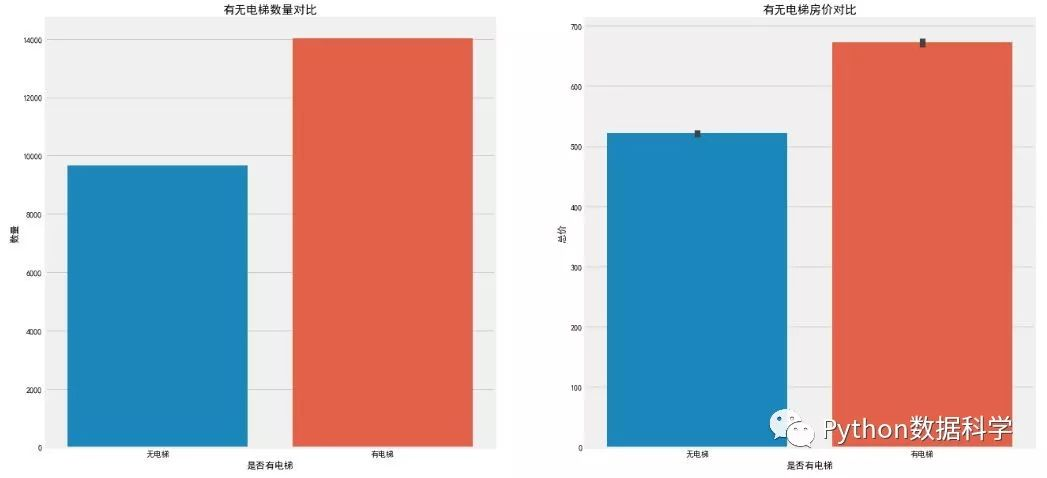结果观察到，有电梯的二手房数量居多一些，毕竟高层土地利用率比较高，适合北京庞大的人群需要，而高层就需要电梯。相应的，有电梯二手房房价较高，因为电梯前期装修费和后期维护费包含内了（但这个价格比较只是一个平均的概念，比如无电梯的6层豪华小区当然价格更高了）。
Year 特征分析
grid = sns.FacetGrid(df, row='Elevator', col='Renovation', palette='seismic',size=4)
grid.map(plt.scatter, 'Year', 'Price')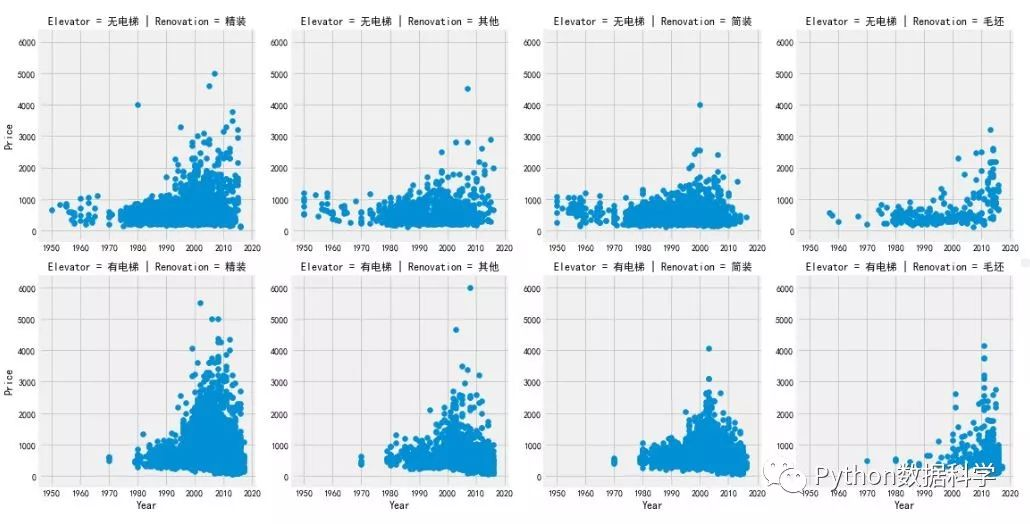在Renovation和Elevator的分类条件下，使用 FaceGrid 分析 Year 特征，观察结果如下：
整个二手房房价趋势是随着时间增长而增长的；  2000年以后建造的二手房房价相较于2000年以前有很明显的价格上涨；  1980年之前几乎不存在有电梯二手房数据，说明1980年之前还没有大面积安装电梯；  1980年之前无电梯二手房中，简装二手房占绝大多数，精装反而很少；
Floor 特征分析
f, ax1= plt.subplots(figsize=(20,5))
sns.countplot(x='Floor', data=df, ax=ax1)
ax1.set_title('房屋户型',fontsize=15)
ax1.set_xlabel('数量')
ax1.set_ylabel('户型')
plt.show()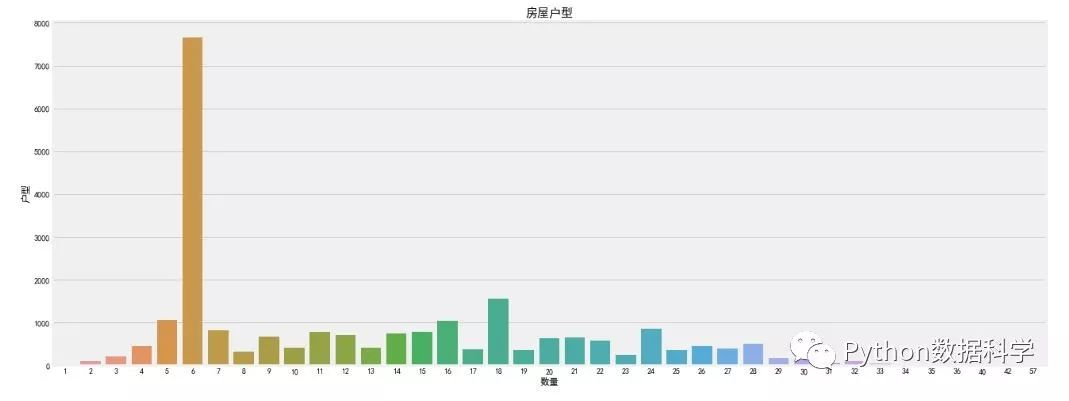可以看到，6层二手房数量最多，但是单独的楼层特征没有什么意义，因为每个小区住房的总楼层数都不一样，我们需要知道楼层的相对意义。另外，楼层与文化也有很重要联系，比如中国文化七上八下，七层可能受欢迎，房价也贵，而一般也不会有4层或18层。当然，正常情况下中间楼层是比较受欢迎的，价格也高，底层和顶层受欢迎度较低，价格也相对较低。所以楼层是一个非常复杂的特征，对房价影响也比较大。
总结
本次分享旨在让大家了解如何用Python做一个简单的数据分析，对于刚刚接触数据分析的朋友无疑是一个很好的练习。不过，这个分析还存在很多问题需要解决，比如：
解决爬虫获取的数据源准确度问题；  需要爬取或者寻找更多好的售房特征；  需要做更多地特征工程工作，比如数据清洗，特征选择和筛选；  使用统计模型建立回归模型进行价格预测；
更多内容会慢慢介绍和分享，敬请期待。我是如何通过Web爬虫找工作的
程序员和 IT 人员不能错过的10个工具
手把手教你如何快速搭建个人网站
用Python告诉你深圳房租有多高？
让你不会装双系统也能用上双系统
用Python分析深圳程序员工资有多高？
Python能用来做什么？以下是Python的三大主要用途
Python爬虫实战（批量采集Stock数据，并保存到Excel中）展开全文Python 爬虫 数据分析 程序员 编程
• 北京二手房信息进行爬取，包括单线程和多线程。 一 单线程 import requests from bs4 import BeautifulSoup m1=[] m2=[] m3=[] for i in range(1,101): urli='https://bj.lianjia.com/ershoufang/pg'+str(i) ...
对北京二手房信息进行爬取，包括单线程和多线程。
一 单线程
import requests
from bs4 import BeautifulSoup
m1=[]
m2=[]
m3=[]
for i in range(1,101):
urli='https://bj.lianjia.com/ershoufang/pg'+str(i)
header = {'user-agent': 'Mozilla/5.0 (Windows NT 6.1; WOW64) AppleWebKit/537.36 (KHTML, like Gecko) Chrome/71.0.3578.98 Safari/537.36'}
htmli=ri.text.encode(ri.encoding).decode()
soupi=BeautifulSoup(htmli,"lxml")
#爬取地址信息
l1i=soupi.findAll('div',attrs={'class':'positionInfo'})
m1i=[i.text for i in l1i]
#爬取房子信息
l2i=soupi.findAll('div',attrs={'class':'houseInfo'})
m2i=[i.text for i in l2i]
#爬取价格信息
l3i=soupi.findAll('div',attrs={'class':'totalPrice'})
m3i=[i.text for i in l3i]
m1=m1+m1i
m2=m2+m2i
m3=m3+m3i
#写入表格
dfs=pd.DataFrame()
dfs['houseInfo']=m2
dfs['positionInfo']=m1
dfs['totalPrice']=m3
dfs.index=range(len(m1))
#导出表格
dfs.to_csv("bj二手房成交.csv")

二 多线程
#多线程爬取房价信息
from time import ctime, sleep
import requests
from bs4 import BeautifulSoup
import pandas as pd
def __init__(self,name,counter):
self.name=name
self.counter=counter
def run(self):
m1=[]
m2=[]
m3=[]
for i in range(self.counter,self.counter+20):
urli='https://bj.lianjia.com/ershoufang/pg'+str(i)
header = {'user-agent': 'Mozilla/5.0 (Windows NT 6.1; WOW64) AppleWebKit/537.36 (KHTML, like Gecko) Chrome/71.0.3578.98 Safari/537.36'}
htmli=ri.text.encode(ri.encoding).decode()
soupi=BeautifulSoup(htmli,"lxml")
l1i=soupi.findAll('div',attrs={'class':'positionInfo'})
m1i=[i.text for i in l1i]
l2i=soupi.findAll('div',attrs={'class':'houseInfo'})
m2i=[i.text for i in l2i]
l3i=soupi.findAll('div',attrs={'class':'totalPrice'})
m3i=[i.text for i in l3i]
m1=m1+m1i
m2=m2+m2i
m3=m3+m3i
dfs=pd.DataFrame()
dfs['houseInfo']=m2
dfs['positionInfo']=m1
dfs['totalPrice']=m3
dfs.index=range(len(m1))
print(dfs,"%s"%ctime())

print("退出主线程")

展开全文• ## Python北京二手房房价数据集分析

千次阅读 热门讨论 2018-10-18 14:20:32
北京二手房的房价跟哪些因素有关呢？ 2.读取数据，理解数据 导入数据分析相关工具包 %matplotlib 为魔法函数，之后的数据可视化过程中，有了它我们就不需要每次都使用plt.show()来显示图表了。 用pandas中的...数据分析
• 利用Python的pandas、pyecharts、sklearn库，对之前从链家网站爬取的北京二手房的数据进行统计和可视化，分析北京二手房价格的影响因素，进而构建随机森林回归模型对北京二手房平均价格进行预测。Python pyecharts sklearn 机器学习 数据分析
• 北京二手房价格预测模型【业务向】2020.8研究背景二手房价格影响因素探索1.指标概述2.北京各地区二手房价格分布3.楼层及朝向对二手房价格的影响4.楼型对北京二手房价格的影响5.装修情况对北京二手房价格的影响6.面积...数据分析 数据可视化 python
• 链家北京二手房交易数据分析 项目背景： 基于之前对机器学习，自然语言处理相关内容的学习，并在kaggle上尝试了泰坦尼克号生还者预测，以及Words Meets Bags of Popcorn两个项目的研究，体会到特征工程的重要性，我...sql 数据分析
• 本篇文章主要是介绍一个北京二手房数据分析的项目，目的是熟悉python数据分析的及可视化的一些常用方法。 数据获取 通过编写python脚本（爬虫）从二手房交易数据网站上获取北京二手房数据集 数据解释 Direction：...
• @北京二手房房价箱线图分析实验 1.添加箱线图节点 在Node Repository的搜索栏中搜索“Conditional Box Plot（local）”，将其拖入工作流编辑器窗口中，命名为“卧室箱线图”。 连接CSV Reader节点和Conditional Box ...
• 爬取北京二手房数据信息python代码： # coding : utf-8 import requests from bs4 import BeautifulSoup as bs from tqdm import tqdm from multiprocessing import Pool import time import os import re import...python 爬虫
• 由于是初次使用Java写爬虫，所以代码有些繁琐，请大家见谅，并能给与指正首先分析链家北京二手房页面，使用360浏览器的审查元素功能，查看源代码，获取查询标签如图一级查询所示，此图标签所获取的是链家北京二手房...java 爬虫
• 此为之前偶尔在社区看到的优秀作业“链家2011-2016北京二手房成交数据分析”，在此为了工作简历上的项目巩固复习练习一次。 环境准备 import numpy as np import pandas as pd import matplotlib.pyplot as ...
• 爬取贝壳找房北京二手房信息数据，并整理成可分析数据 数据获取 （修正后数据链接：链接：https://pan.baidu.com/s/1C3_eseM-wjW3mo-WUvgCGw 提取码：73iw） 1.爬虫 从贝壳找房爬取北京二手房最新数据，...爬虫
• 目标：爬取安居客网站上前10页北京二手房的数据，包括二手房源的名称、价格、几室几厅、大小、建造年份、联系人、地址、标签等。 网址为：https://beijing.anjuke.com/sale/ BeautifulSoup官网：...python
• 数据科学俱乐部中国数据科学家社区本篇将继续上一篇数据分析用Python分析北京二手房房价之后进行数据挖掘建模预测，这两部分构成了一个简单的完整项目。结合两篇文章通过数据分...
• 最近呢，对链家平台上的北京二手房数据做了个可视化分析，对目前北京的二手房交易情况有了个大致了解，最终得到一个很实在的结论：奋斗一辈子也买不到一个厕所这句话不是骗人的，是真的；关于具体分析内容客官请看...
• 本项目以研究北京二手房房价为目的，通过Scrapy框架爬取链家网站的二手房房源信息，对其进行基本的数据分析及可视化，并利用决策树算法对未来房价进行预测，最后，可视化模型的学习曲线，观察是否出现过拟合问题。...数据挖掘 爬虫 数据分析 可视化
• 之前我们爬取了贝壳找房上的北京二手房信息，具体可以查看python爬取贝壳找房之北京二手房源信息，现在我们针对获取的数据进行分析及可视化的展示，本文代码和数据均存放在github上 数据预处理 由于我们爬取的数据...python 数据分析
• 最近呢，对链家平台上的北京二手房数据做了个可视化分析，对目前北京的二手房交易情况有了个大致了解，最终得到一个很实在的结论：奋斗一辈子也买不到一个厕所这句话不是骗人的，是真的；关于具体分析内容请看下文 ...
• day1:学习python抓取数据–链家北京二手房数据 最近在学习用Python进行数据分析、机器学习，使用数据集效果不错，想用一些实际数据看一下效果，于是想到用Python尝试抓取一些数据。 实验目的：学习Python爬取数据...Python 爬虫
• 本次实战项目的主要目的是分析北京二手房房价，项目源自博文：入门Python数据分析最好的实战项目（一）和入门Python数据分析最好的实战项目（二）。本篇文章仅记录博主在学习过程中的思路。 数据分析 首先我们要对...
• 商业大数据线性回归实验:北京二手房房价实验步骤（上） 实验要求 （1）使用北京二手房房价.csv文件，创建一个工作流。 （2） 用北京二手房房价.csv文件中的数据生成单位面积房价直方图、内部因素的单位面积房价箱...
• 同学们好，完成后将计分练习的整个项目提交，包括源码与生成的数据和图片压缩！...在链家二手房平台爬取自己家乡或者北京的3~4个区域的二手房信息，处理后保存为data.csv，并探究不同的区二手房总价数据！python...